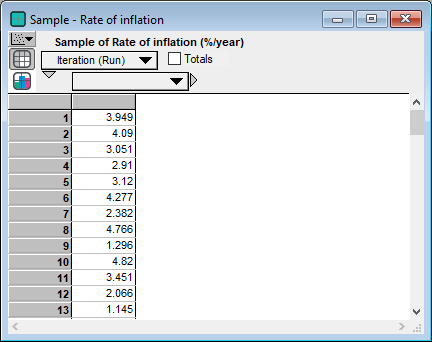# Uncertainty views

Every variable has a certain or deterministic value, which we term its mid value. Some variables -- chance variables and variables influenced by chance variables -- can also have an uncertain or probabilistic value, which we term its prob value. A mid value is computed using the mid value of each variable it depends on or the median of any probability distribution. The mid value of a result is not necessarily the median of its probability distribution, but usually close.

The Result window offers eight views, seven of which being uncertainty views, including the mid value (which is not uncertain) and seven ways to display a prob value. You can select the uncertainty views from a menu in the top-left corner of a Result window. Or you can select a variable, and select an uncertainty view option from the Result menu.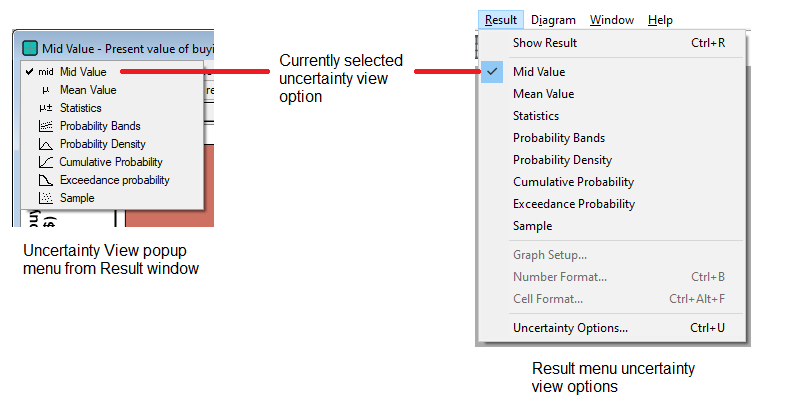The checkmark indicates the currently selected view.

Here we illustrate each uncertainty view using the chance variable, Rate_of_inflation, defined as a normal distribution with a mean of 2.5 and a standard deviation of 1:

Chance Rate_of_inflation := Normal(2.5, 1)

## Mid value

The mid value is the deterministic value, computed by using the median instead of any input probability distribution. It is computed very quickly compared to uncertain values. It is the only option available for a variable that is not probabilistic.Tip
A mid value is much faster to compute than a prob(abilistic) value, since it doesn’t use Monte Carlo simulation to compute a probabilistic sample. It is often useful to look first at the mid value of a variable as a quick sanity check. Then you might select an uncertainty view, which causes its prob value to be computed if it has not already been cached.

## Mean value

An estimate of the mean (or expected value) of the uncertain value, based on the random (Monte Carlo) sample.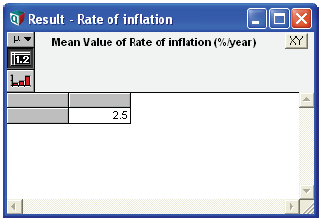Tip
The mean and the other uncertainty views below are estimates based on the Monte Carlo (or Latin hypercube) sample. The precision of these estimates depends on the sample size and the sampling method. A larger sample size gives higher precision and takes more time and memory to compute. You can modify the sample size and sampling method in the Uncertainty Setup dialog from the Result menu.

## Statistics

A table of statistics of the uncertain value, usually, the minimum, median, mean, maximum, and standard deviation, estimated from the random sample. You can select which statistics to show in the Statistics tab of the Uncertainty Setup dialog from the Result menu.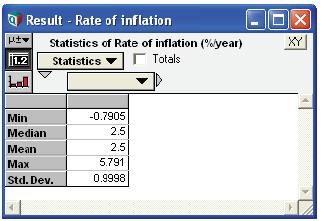## Probability bands

An array of percentiles (fractiles) estimated from the random sample. By default, it shows the 5%, 25%, 50%, 75%, and 95%iles. You can select which percentiles to show in the Probability Bands tab of the Uncertainty Setup dialog from the Result menu.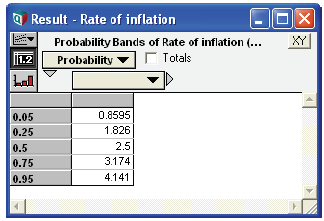## Probability density

Select probability density to display the uncertain distribution as a probability density function (PDF).

For a probability density function, it plots values of the quantity over the X (usually horizontal) axis, and probability density on the Y (vertical axis). Probability density shows the relative probability of different values. High values show probable regions; low values show less probable regions. The peak is the mode, the most probable value. If the density is zero, it is certain that the quantity will not have values in that range.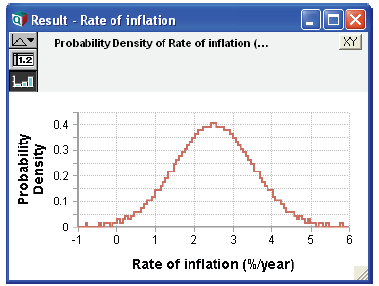Probability mass function: If you select Probability density for a discrete variable, it displays the variable as a probability mass function (PMF) in a bar graph with the height of each bar indicating the probability of that value.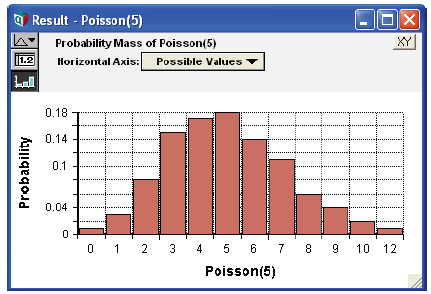Usually, it figures out whether to use a probability density or mass function. Rarely, you might need to tell it the domain is discrete. See The domain attribute and discrete variables Is the quantity discrete or continuous?, and Probability density and mass graphs for more.

## Cumulative probability

The cumulative probability distribution (CDF) plots the possible values of the uncertain quantity along the X (usually horizontal) axis. The Y value (usually height) of the graph at each value of X shows the probability that the quantity is less than or equal to that X value. The CDF must start at a probability of 0 on the extreme left and increase to a probability of 1 on the extreme right, never decreasing.The steeper the curve, the more likely the quantity will have a value in that region. The PDF is the slope (first derivative) of the CDF. Conversely, the CDF is the cumulative integral of the PDF.

## Exceedance probability distribution

The exceedance probability distribution (XPD) is the complement of the Cumulative probability distribution (CDF) -- i.e. XPD = 1 - CDF. It plots the probability that uncertain quantity X will exceed each value along the X axis. It must start at a probability of 1 on the extreme left and decrease to a probability of 0 on the extreme right, never increasing.

The steeper the curve, the more likely the quantity will have a value in that region. The PDF is the inverse slope (first derivative) of the XPD.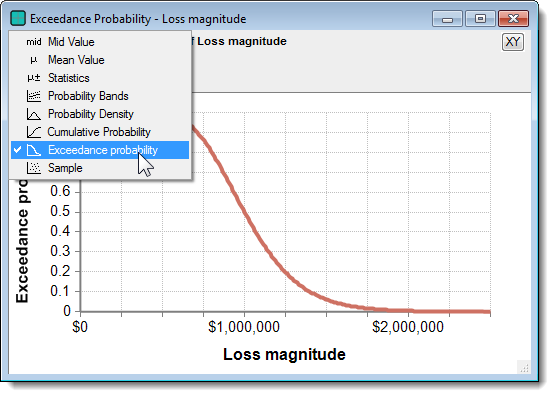## Sample

A sample is an array of the random values from the distribution generated by the Monte Carlo sampling process. The sample is the underlying form used to represent each uncertain quantity. All the other uncertainty views use statistics estimated from the sample. The sample view gives more detail than you usually want. You will likely want to view it mainly when verifying or debugging a model.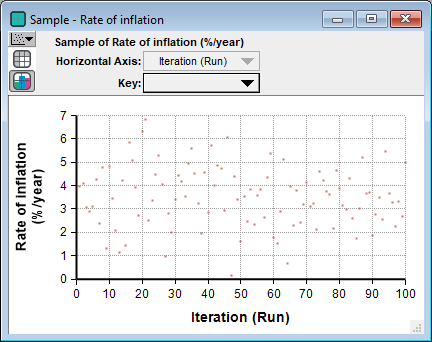Like any other graph, you can display a sample as a table by clickingto see the underlying numerical values.